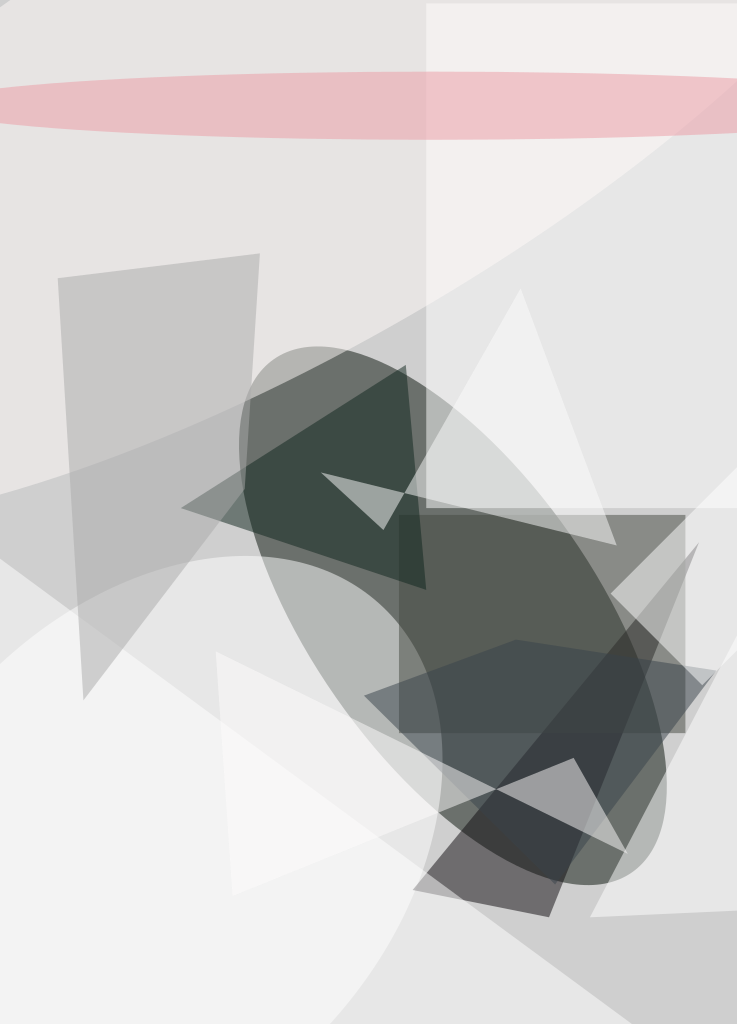Some progress has been made in a continuing effort to develop mathematical models of the behaviors of multi- agent systems known in biology, economics, and sociology (e.g., systems ranging from single or a few biomolecules to many interacting higher organisms). This effort at an earlier stage was reported in "Characteristics of Dynamics of Intelligent Systems" (NPO- 21037), NASA Tech Briefs, Vol. 26, No. 12 (December 2002), page 48.

To recapitulate from the cited prior article: Living systems can be characterized by nonlinear evolution of probability distributions over different possible choices of the next steps in their motions. One of the main challenges in mathematical modeling of living systems is to distinguish between random walks of purely physical origin (for instance, Brownian motions) and those of biological origin. Following a line of reasoning from prior research, it has been assumed, in the present development, that a biological random walk can be represented by a nonlinear mathematical model that represents coupled mental and motor dynamics incorporating the psychological concept of reflection or self-image. The nonlinear dynamics impart the lifelike ability to behave in ways and to exhibit patterns that depart from thermodynamic equilibrium. Reflection or self-image has traditionally been recognized as a basic element of intelligence.

The nonlinear mathematical models of the present development are denoted self-supervised dynamical systems. They include (1) equations of classical dynamics, including random components caused by uncertainties in initial conditions and by Langevin forces, coupled with (2) the corresponding Liouville or Fokker-Planck equations that describe the evolutions of probability densities that represent the uncertainties. The coupling is effected by fictitious information-based forces, denoted supervising forces, composed of probability densities and functionals thereof.

The equations of classical mechanics represent motor dynamics — that is, dynamics in the traditional sense, signifying Newton's equations of motion. The evolution of the probability densities represents mental dynamics or self-image. Then the interaction between the physical and metal aspects of a monad is implemented by feedback from mental to motor dynamics, as represented by the aforementioned fictitious forces. This feedback is what makes the evolution of probability densities nonlinear. The deviation from linear evolution can be characterized, in a sense, as an expression of free will.

It has been demonstrated that probability densities can approach prescribed attractors while exhibiting such patterns as shock waves, solitons, and chaos in probability space. The concept of self-supervised dynamical systems has been considered for application to diverse phenomena, including information-based neural networks, cooperation, competition, deception, games, and control of chaos. In addition, a formal similarity between the mathematical structures of self-supervised dynamical systems and of quantum-mechanical systems has been investigated.

This work was done by Michail Zak of Caltech for NASA's Jet Propulsion Laboratory. For further information, access the Technical Support Package (TSP) free on-line at www.techbriefs.com/tsp under the Information Sciences category. NPO-30634.

##### This Brief includes a Technical Support Package (TSP).###### Self-Supervised Dynamical Systems